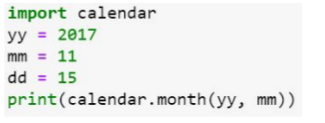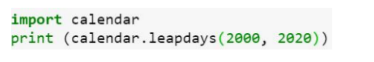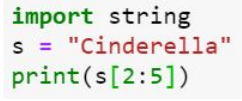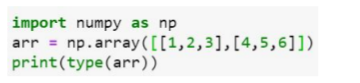# NPTEL » The Joy Of Computing Using Python 2021The Joy of Computing using Python This course is an introduction to programming and problem-solving in Python. It does not assume any prior knowledge of programming. A fun-filled whirlwind tour of 30 hrs, covering everything you need to know to fall in love with the most sought after skill of the 21st century. The course brings programming to your desk with anecdotes, analogies, and illustrious examples. Turning abstractions to insights and engineering to art, the course focuses primarily to inspire the learner’s mind to think logically and arrive at a solution programmatically.

The Joy of Computing using Python is a MOOC based course that is 12 weeks in duration and can fulfill the criteria of 4 credits in a year. You can visit the NPTEL SWAYAM platform and register yourself for the course. This course is brought to you by Prof.Sudarshan Iyengar has a Ph.D. from the Indian Institute of Science and is currently working as an assistant professor at IIT Ropar and has been teaching this course for the past 4 years.

Who Can JoinAny discipline  PREREQUISITES: Minimum: 10th standard/high school Ideal: Computer Architecture, Basic OS and Networking concepts

INDUSTRY SUPPORT: All industries

CRITERIA TO GET A CERTIFICATE

Average assignment score = 25% of the average of the best 6 assignments out of the total 8 assignments given in the course.
Exam score = 75% of the proctored certification exam score out of 100

Final score = Average assignment score + Exam score

Students will be eligible for CERTIFICATE ONLY IF AVERAGE ASSIGNMENT SCORE >=10/25 AND EXAM SCORE >= 30/75. If any of the 2 criteria are not met, the student will not get the certificate even if the Final score >= 40/100.

### The Joy Of Computing Using Python Quiz Assignment Week 12 Answers:-

Contents

Q1. Which of the following is true about the web graph used for performing Google page ranking?

Answer:- B – nodes are the web pages and edges are the hyperlinks

Q2. In page ranking, the most impressive person is the person liked by maximum number of people.

Q3. What is not true about page ranking algorithm?

Answer:- D – high ranked node is the one with maximum hyperlink

Q4. In page ranking algorithm

Answer:- A – we always begin ranking from the first node.

Q5. In Barbell graph() function of Networkx

Answer:- B – the first parameter represents number of nodes in the two communities and the second parameter represents number of nodes in-between the communities

Q6.What is the type of the following graph?

Q7. Which of the following graph represent a Wheel graph of 5 nodes?

Q8. What is the next step in page ranking algorithm, if the current node in the walk is a sink ?

Answer:- B – the next node is selected randomly from the given set of nodes present in the graph

Q9. Which of the following is a directed network?

Answer:- D – All of the above

Q10. Which of the following python function will return random floating point number between 0 and 1?

### The Joy Of Computing Using Python Quiz Assignment Week 11 Answers:-

Q1. The python library selenium is used for which of the following concepts

Q2. Which of the following is true about Browser automation?

Answer:- D – All of the above

Q3. The python function for converting a number into a string is

Q4. Which of the following is the python library for setting the timezone?

Q5. Which of the following code snippet will print today’s date?

Answer:- C – Both A and B

Q6. Predict the output:Q7. What does the following code print?Answer:- A – Number of leapdays between the specified years

Q8. What does the python function: calendar.weekday(year, month, day) return if the weekday is Friday?

Q9. What is the return value of the following python function : datetime.datetime.utcnow()

Q10. Which of the following is the correct code to find whether a given year is a leap year or not?

### The Joy Of Computing Using Python Quiz Assignment Week 10 Answers:-

Q1. The game ”FLAMES” represents which of the following mathematics question?

Q2. Predict the output of the following code

Q3. Predict the output of the following code

Q4. What does the following code snippet in python print?Q5. In python, the default value of start and end index of list slicing are which of the following options?

Answer:- C – 0, length of the list -1

Q6. Which of the following is not a functionality of string in python?

Q7. Predict the outputQ8. Which of the following code snippet will print transpose of the matrix a?

Q9. Which of the following will print column sum of the matrix a?

Q10. Image compression is always a lossy compression.

### The Joy Of Computing Using Python Quiz Assignment Week 9 Answers:-

Q1. Which of the following is not true about Stylometry Analysis?

Answer:- C – any two people may have same vocabulary

Q2. An author’s stylic signature can be analysed by which of the following method(s)?

Answer:- D – All of the above

Q3. What is the output of the following code?

Answer:- B – [’Have nice day, my friend!!!’, ’Programming in Python is fun’]

Q4. What is the output of the following code?

Q5. Strings in python can be created using

Answer:- E – A, B and C

Q6. Networkx in python is used for which of the following operation(s)?

Answer:- D – All of the above

Q7. Which of the following will generate a complete graph in python using Networkx package?

Answer:- B – Graph = nx.gnp random graph(25,1.0)

Q8. Degree of separation of a complete graph with n nodes is always

Q9. Which of the following is true about six degrees of seperation?

Answer:- C – the average degree of separation of the nodes in the network is 6

Q10. Which of the following method will return the RBG value of a pixel in python?

### The Joy Of Computing Using Python Quiz Assignment Week 8 Answers:-

Q1. Which of the following code snippet will create a tuple in python?

Q2. Which of the following is not true about tuples in python?

Answer:- C- Tuple supports item deletion

Q3. What is the output of the following code snippet in python?name =(’kiran’,’bhushan’,’madan’)print (name[-1])

Q4. What is the output of the following code?

Answer:- A – the program stops when the number entered matches with the random number generated

Q5. What does the following program plot?

Answer:- B – Plots the number of times the given input matches with the random number generated

Q6. In image processing using python what is the acronym of PIL?

Answer:- D – Python Imaging Library

Q7. What does the following code snippet in python compute?

Answer:- B – searches for text2 in text1

Q8. Which of the following code will convert the uppercase letters of the given string into lower case and prints the converted string?

Answer:- C – both A and B

Q9. Which of the following is the platform for building Python programs to work with sentiment analysis of human language data?

Answer:- B – NLTK: Natural Language Toolkit

Q10. Sentiment analysis involves working with whether  __________

Answer:- D – a piece of information is positive or negative

### The Joy Of Computing Using Python Quiz Assignment Week 7 Answers:-

Q1. Which of the following is/are uses of functions?

Answer:- D- All of the above

Q2. In Snakes and Ladders game the least number of times a player has to roll a die with the following ladder positions is ____________ladders = { 3: 20, 6: 14, 11: 28, 15: 34, 17: 74, 22: 37, 38: 59, 49: 67, 57: 76, 61: 78, 73: 86, 81: 98, 88: 91 }

Q3. Which of the following is the end point of the game Snakes and Ladder?

Answer:- C – both A and B are the possibilities of the game to end

Q4. What is the output of the following spiralprint python function?

Answer:- B – 1 2 3 4 5 6 12 18 17 16 15 14 13

Q5. Which of the following code snippet will draw a star?

Q6. Which of the following code snippet will draw a Hexagon?

Q7. What is the output of the following code?

Q8. In a file with extension csv what does csv mean?

Answer:- D – comma separated value

Q9. which of the following library has to be imported to plot the route map using GPS locations in python?

Q10.Which of the following library moves the turtle backward?

Answer:- D -All of the above

### The Joy Of Computing Using Python Quiz Assignment Week 6 Answers:-

Q1. In Caesar cipher, the mediator needs to make maximum of how many trails to break the code?

Q2. What is the result of the following code if the input is COMPUTING?

``````result = ""
text = input()
shift = 4
for i in range (len(text)):
char = text[i]
if (char.isupper()):
result += chr((ord(char) + shift-65) % 26 + 65)
else:
result += chr((ord(char) + shift 97) % 26 + 97)``````

Q3. Which of the following is TRUE about MIN-MAX strategy?

Answer:- A – Maximise the chances of your winning and minimize the changes of the opponent winning

Q4. What is the output of the following code?

``````num1 = int(input())
num2 = int (input())
i = 1 %3D
while(i <= num1 and i <= num2):
if(num1 % i == 0 and num2 % i == e):
output i
i = i + 1``````

Answer:- A – Greatest common factor of num1 and num2

Q5. What does the following python code compute?

``````def xyz(a, b):
if a == 0 or b == 0: return e
if b == 1:
return a
if a == 1:
return b
return a + xyz(a, b - 1)``````

Answer:- C – product of a and b

Q6. Which of the following is not true about recursion?

Answer:- C – The speed of a program using recursion is faster than the speed of its non-recursive equivalent

Q7. Which of the following is the optimal code among the given codes using recursive binary search?

Q8. What is the result of the following recursive function call?

Q9. What is the output of the following python code?

Q10. A program can be written using recursive function only if it can be recursively defined.

### The Joy Of Computing Using Python Quiz Assignment Week 5 Answers:-

Q1. Let marks scored be a dictionary of the items given below:

``marks_scored = { }marks_scored[’maths’]= 80marks_scored[’science’]=90marks_scored[’english’]=85marks_scored[’social’]=95``

Which of the following operation will print the items of the dictionary?

Q2. Which of the following operation on the dictionary marks_scored in Question 1 will remove a specified key and return the corresponding value?

Q3. Speech recognition does not work on .wav extension files

Q4. What are the items in the following dictionary :

``````Dictionary = {x: x*x for x in range(11) if x % 2 == 0 }
print(Dictionary)``````

Answer:- B – Dictionary of even numbers and their squares

Q5. In the game ”Rock, Paper and Scissor”, if player one enters 456 and player two enters 684 with their secret bits 0 and 2 respectively, then the expected outcome of the game would be ______

Q6. What is the output of the following code:

``````
Ajay
sem : 3
roll_no : 1
total_marks : 85
Shwetha
sem : 3
roll_no : 2
total_marks : 90
``````

Q7. Binary search can be applied on any list of random elements

Q8. Which of the following is true about bubble sort?

Answer:- C – In each iteration every consecutive pairs of the unsorted list are compared

Q9. Which are the given statements precisely explains the action of the given code?

Answer:- B – rolls the dices as long as the input is ’y’ or the sum of their face values is 12

Q10. What will be the output of the following code?

Answer:- C – 2 3 01

### The Joy Of Computing Using Python Quiz Assignment Week 4 Answers:-

Q1. A magic square is an n ×n matrix in which

Answer:- D- All of the above

Q2. For any magic square of n × n, the magic number M is given by

Answer:- A – n (n^2 + 1) / 2

Q3. Assuming that num is always a 2-digit number, what is the output of the following code?

Q4. In a double game each pair of cards will have

Answer:- B – Only one symbol in common

Q5. The minimum number of people required to guarantee that at least two people will have their birthdays falling on the same day of a non-leap year is

Q6. What does the following code snippet in python compute?

``````		num = int(input())
for i in range(1, 11):
print(num*i)``````

Answer:- B- Multiplication table of num

Q7. Which of the following will print all prime numbers in an interval?

Q8. Which of the following method in python choses a movie from the list of movie names given below?

movies =[“zindagi” , ” chinatown ” , “darr” , ” 3idiots ” , “sixthsense” , “speed” , “avtaar”]